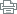skip navigation

This page is designed for modern browsers. You will have a better experience with a better browser.# Math Department Goals & Assessment

Math Dept. Goals:

Students will demonstrate:

1. Mathematical correctness and proficiency.
2. Proficiency in mathematical language and notation
3. The ability to interpret real world situations and apply mathematical concepts.

Math Dept. Program Level Outcomes (PLO's):

Students will be able to:

1. calculate arithmetic, algebraic, geometric, spatial, and statistical quantities using appropriate technology.
2. estimate arithmetic, algebraic, geometric, spatial, and statistical solutions.
3. solve arithmetic, algebraic, geometric, spatial, and statistical expressions, equations, functions, and problems using appropriate technology.
4. represent mathematical information numerically, symbolically, graphically, verbally, and visually using appropriate technology.
5. interpret mathematical and statistical models such as formulas, functions, graphs, tables, and schematics, drawing conclusions and making inferences based on those models.
6. develop mathematical and statistical models such as formulas, functions, graphs, tables, and schematics using appropriate technology.
7. communicate mathematical theories and ideas clearly and concisely to others in the oral and written form.

The Math Department regularly takes a look at its students' success and tries to modify its offerings accordingly.  Each semester we assess our student learning outcomes (SLO's) and review the results from the previous term.  We use success and retention rate data to analyze the strengths and weaknesses of our course offerings, modifying our teaching strategies and offerings accordingly.

Student Learning Outcomes (SLO's) for each of our math courses can be found HERE (updated April 2014).

SLO Trends

Summaries of SLO Assessments, by term:

2014: Spring, Summer

2013: Spring, Summer, Fall

2012: Spring, Summer, Fall

2011: Fall

PLO Trends

Summaries of PLO Assessments, by term:

2014: Spring

2013: Spring, Fall